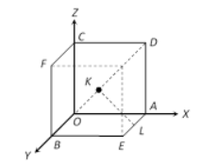# Program to find the shortest distance between diagonal and edge skew of a Cube

Given an integer A, denoting the length of a cube, the task is to find the shortest distance between the diagonal of a cube and an edge skew to it i.e. KL in the below figure.Examples :

Input: A = 2
Output: 1.4142
Explanation:
Length of KL = A / ?2
Length of KL = 2 / 1.41 = 1.4142

Input: A = 3
Output: 2.1213

Approach: The idea to solve the problem is based on the following mathematical formula:

Let’s draw a perpendicular from K to down face of cube as Q.
Using Pythagorean Theorem in triangle QKL,

KL2 = QK2 + QL2

l2 = (a/2)2 + (a/2)2

l2 = 2 * (a/2)2

l = a / sqrt(2)

Below is the implementation of the above approach:

## C++

 `// C++ program for the above approach`   `#include ` `using` `namespace` `std;`   `// Function to find the shortest distance` `// between the diagonal of a cube and` `// an edge skew to it` `float` `diagonalLength(``float` `a)` `{` `    ``// Stores the required distance` `    ``float` `L = a / ``sqrt``(2);`   `    ``// Print the required distance` `    ``cout << L;` `}`   `// Driver Code` `int` `main()` `{` `    ``// Given side of the cube` `    ``float` `a = 2;`   `    ``// Function call to find the shortest` `    ``// distance between the diagonal of a` `    ``// cube and an edge skew to it` `    ``diagonalLength(a);`   `    ``return` `0;` `}`

## Java

 `// Java program for the above approach`   `class` `GFG {`   `    ``// Function to find the shortest` `    ``// distance between the diagonal of a` `    ``// cube and an edge skew to it` `    ``static` `void` `diagonalLength(``float` `a)` `    ``{` `        ``// Stores the required distance` `        ``float` `L = a / (``float``)Math.sqrt(``2``);`   `        ``// Print the required distance` `        ``System.out.println(L);` `    ``}`   `    ``// Driver Code` `    ``public` `static` `void` `main(String[] args)` `    ``{` `        ``// Given side of the cube` `        ``float` `a = ``2``;`   `        ``// Function call to find the shortest` `        ``// distance between the diagonal of a` `        ``// cube and an edge skew to it` `        ``diagonalLength(a);` `    ``}` `}`

## Python3

 `# Python3 program for the above approach`   `from` `math ``import` `sqrt`   `# Function to find the shortest ` `# distance between the diagonal of a ` `# cube and an edge skew to it` `def` `diagonalLength(a):` `  `  `    ``# Stores the required distance` `    ``L ``=` `a ``/` `sqrt(``2``)`   `    ``# Print the required distance` `    ``print``(L)`     `# Given side of the cube` `a ``=` `2`   `# Function call to find the shortest ` `# distance between the diagonal of a ` `# cube and an edge skew to it` `diagonalLength(a)`

## C#

 `// C# program for the above approach`   `using` `System;` `class` `GFG {`   `    ``// Function to find the shortest` `    ``// distance between the diagonal of a` `    ``// cube and an edge skew to it` `    ``static` `void` `diagonalLength(``float` `a)` `    ``{` `        ``// Stores the required distance` `        ``float` `L = a / (``float``)Math.Sqrt(2);`   `        ``// Print the required distance` `        ``Console.Write(L);` `    ``}`   `    ``// Driver Code` `    ``public` `static` `void` `Main()` `    ``{` `        ``// Given side of the cube` `        ``float` `a = 2;`   `        ``// Function call to find the shortest` `        ``// distance between the diagonal of a` `        ``// cube and an edge skew to it` `        ``diagonalLength(a);` `    ``}` `}`

## PHP

 ` `

## Javascript

 ``

Output:

`1.41421`

Time Complexity: O(1)
Auxiliary Space: O(1)

Feeling lost in the world of random DSA topics, wasting time without progress? It's time for a change! Join our DSA course, where we'll guide you on an exciting journey to master DSA efficiently and on schedule.
Ready to dive in? Explore our Free Demo Content and join our DSA course, trusted by over 100,000 geeks!

Previous
Next